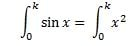# Area of y = sin x is equal to y = x^2, find k

• Knight226

## Homework Statement

If the area under y = sin x is equal to the area under y = x^2 between x = 0 and x = k, then k = :

## The Attempt at a Solution

I do not have any idea how to do this problem. So I asked my friends for help, but their methods are different. One of my friend says that he assumes k is 1 because of the graph of sin x. The other friend uses the following method:That method gives the result of about 1.1

Now… my question is… how should one know k is the upper value? I mean, in the graph of sin x, there is a -1 as well. That will make 0 the upper value and -1 the lower bound value (wouldn’t it?)

The mathematical method sounds reasonable because the areas are equal. But, I am not quite sure how to continue from that equation… she said she wasn’t sure about that equation so didn’t explain to me how to continue the problem.

My biggest question is, are they doing the problem correctly and how should I approach this problem?